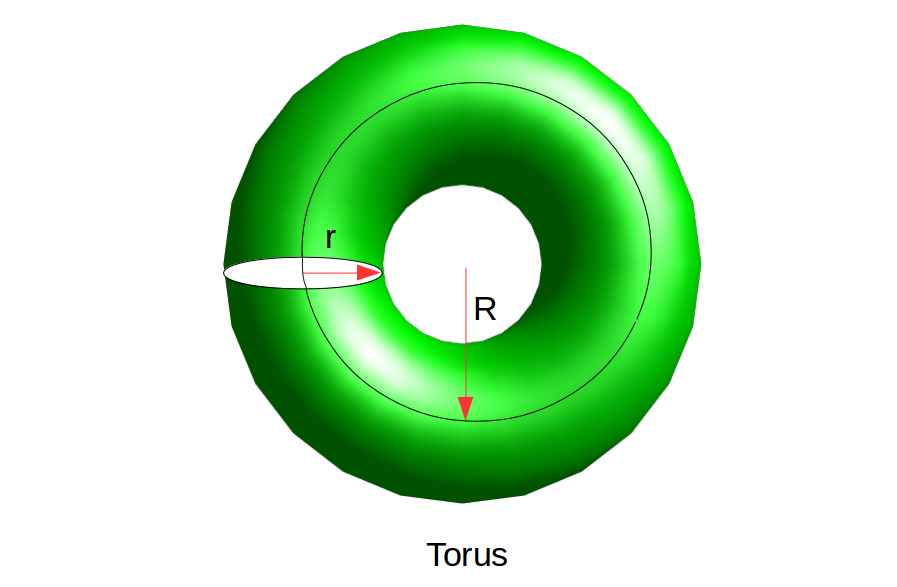# Calculate volume and surface area of TorusTorus

Property:

2. It is not a polyhedron
3. It has no vertices or edges
• Surface Area
The surface area of a Torus is given by the formula –

`Surface Area = 4 × Pi^2 × R × r`

Where r is the radius of the small circle and R is the radius of bigger circle and Pi is constant Pi=3.14159.

• Volume
The volume of a cone is given by the formula –

`Volume = 2 × Pi^2 × R × r^2`

Where r is the radius of the small circle and R is the radius of bigger circle and Pi is constant Pi=3.14159.

Examples:

```Input : r=3, R=7
Output :
Volume: 1243.568195
Surface: 829.045464
```

## C++

 `// C++ program to calculate volume ` `// and surface area of Torus ` `#include ` `using` `namespace` `std; ` ` `  `int` `main() ` `{ ` `    ``// radus of inner circle ` `    ``double` `r = 3; ` ` `  `    ``// distance from origin to center of inner circle ` `    ``// radius of black circle in figure ` `    ``double` `R = 7; ` ` `  `    ``// Value of Pi ` `    ``float` `pi = (``float``)3.14159; ` `    ``double` `Volume = 0; ` `    ``Volume = 2 * pi * pi * R * r * r; ` `    ``cout<<``"Volume: "``<

## C

 `// C program to calculate volume  ` `// and surface area of Torus ` `#include ` `int` `main() ` `{ ` `    ``// radus of inner circle ` `    ``double` `r = 3; ` ` `  `    ``// distance from origin to center of inner circle ` `    ``// radius of black circle in figure ` `    ``double` `R = 7; ` ` `  `    ``// Value of Pi ` `    ``float` `pi = (``float``)3.14159; ` `    ``double` `Volume = 0; ` `    ``Volume = 2 * pi * pi * R * r * r; ` `    ``printf``(``"Volume: %f"``, Volume); ` ` `  `    ``double` `Surface = 4 * pi * pi * R * r; ` `    ``printf``(``"\nSurface: %f"``, Surface); ` `} `

## Java

 `// Java program to calculate volume  ` `// and surface area of Torus ` `class` `Test { ` ` `  `    ``public` `static` `void` `main(String args[]) ` `    ``{ ` ` `  `        ``// radius of inner circle ` `        ``double` `r = ``3``; ` ` `  `        ``// distance from origin to center of inner circle ` `        ``// radius of black circle in figure ` `        ``double` `R = ``7``; ` ` `  `        ``// Value of Pi ` `        ``float` `pi = (``float``)``3.14159``; ` `        ``double` `Volume = ``0``; ` `        ``Volume = ``2` `* pi * pi * R * r * r; ` `        ``System.out.printf(``"Volume: %f"``, Volume); ` ` `  `        ``double` `Surface = ``4` `* pi * pi * R * r; ` `        ``System.out.printf(``"\nSurface: %f"``, Surface); ` `    ``} ` `} `

## Python3

 `# Python3 program to calculate volume ` `# and surface area of Torus ` `# radus of inner circle ` `r ``=` `3` ` `  `# distance from origin to center of inner circle ` `# radius of black circle in figure ` `R ``=` `7` ` `  `# Value of Pi ` `pi ``=` `3.14159` `Volume ``=` `(``float``)(``2` `*` `pi ``*` `pi ``*` `R ``*` `r ``*` `r); ` `print``(``"Volume: "``, Volume); ` `Surface ``=` `(``float``)(``4` `*` `pi ``*` `pi ``*` `R ``*` `r); ` `print``(``"Surface: "``, Surface); `

## C#

 `// C# program to calculate volume  ` `// and surface area of Torus  ` `using` `System; ` ` `  `class` `GFG  ` `{  ` `     `  `// Driver Code ` `public` `static` `void` `Main()  ` `{  ` ` `  `    ``// radius of inner circle  ` `    ``double` `r = 3;  ` ` `  `    ``// distance from origin to center  ` `    ``// of inner circle radius of black ` `    ``// circle in figure  ` `    ``double` `R = 7;  ` ` `  `    ``// Value of Pi  ` `    ``float` `pi = (``float``)3.14159;  ` `    ``double` `Volume = 0;  ` `    ``Volume = 2 * pi * pi * R * r * r;  ` `    ``Console.WriteLine(``"Volume: {0}"``, Volume);  ` ` `  `    ``double` `Surface = 4 * pi * pi * R * r;  ` `    ``Console.WriteLine(``"Surface: {0}"``, Surface);  ` `}  ` `}  ` ` `  `// This code is contributed by Soumik `

## PHP

 ` `

Output:

```Volume: 1243.568195
Surface: 829.045464
```

Attention reader! Don’t stop learning now. Get hold of all the important DSA concepts with the DSA Self Paced Course at a student-friendly price and become industry ready.

My Personal Notes arrow_drop_upCheck out this Author's contributed articles.

If you like GeeksforGeeks and would like to contribute, you can also write an article using contribute.geeksforgeeks.org or mail your article to contribute@geeksforgeeks.org. See your article appearing on the GeeksforGeeks main page and help other Geeks.

Please Improve this article if you find anything incorrect by clicking on the "Improve Article" button below.

Article Tags :
Practice Tags :

Be the First to upvote.

Please write to us at contribute@geeksforgeeks.org to report any issue with the above content.